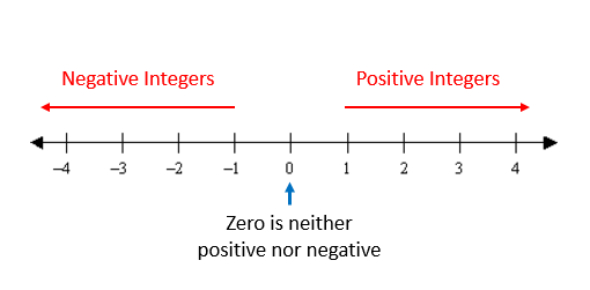# Integer Quiz: Math GK Test!

Approved & Edited by ProProfs Editorial Team
The editorial team at ProProfs Quizzes consists of a select group of subject experts, trivia writers, and quiz masters who have authored over 10,000 quizzes taken by more than 100 million users. This team includes our in-house seasoned quiz moderators and subject matter experts. Our editorial experts, spread across the world, are rigorously trained using our comprehensive guidelines to ensure that you receive the highest quality quizzes.
| By Naveen24t
N
Naveen24t
Community Contributor
Quizzes Created: 3 | Total Attempts: 10,373
Questions: 8 | Attempts: 1,869Settings.

• 1.

### The product of two numbers is 486, If the LCM is 54,then HCF is

• A.

18

• B.

27

• C.

9

• D.

1

C. 9
Explanation
The product of two numbers is 486 and the LCM is 54. To find the HCF, we need to find the largest common factor of the two numbers. Since the LCM is 54, it means that both numbers are divisible by 54. So, the HCF must also be a factor of 54. The only option that is a factor of 54 is 9. Therefore, the HCF is 9.

Rate this question:

• 2.

### The nearest number to 107 which is divisible by 4.

• A.

102

• B.

106

• C.

104

• D.

108

D. 108
Explanation
108 is the nearest number to 107 that is divisible by 4 because it is greater than 107 and the smallest multiple of 4 that is greater than 107.

Rate this question:

• 3.

### The integers lie between 0 and -4.

• A.

-4,-5,-6

• B.

-1,-2,-3

• C.

0,-1,-2,-3

• D.

-1,-2,-3,-4

B. -1,-2,-3
Explanation
The integers lie between 0 and -4, which means they are negative numbers greater than -4 and less than 0. The given answer of -1, -2, -3 satisfies this condition as they are negative numbers greater than -4 and less than 0.

Rate this question:

• 4.

### The increasing order of 0,5,-7.

• A.

-7,0,5

• B.

-7,5,0

• C.

5,0,-7

• D.

5,-7,0

A. -7,0,5
Explanation
The correct answer is -7,0,5 because it follows the increasing order. The numbers are arranged in ascending order from left to right, starting with the smallest number (-7), followed by 0, and then 5.

Rate this question:

• 5.

### The largest negative integer is?

• A.

Infinite

• B.

-1000

• C.

-1

• D.

0

C. -1
Explanation
The largest negative integer is -1 because it is the smallest number that is less than 0. Any number smaller than -1 would be a larger negative integer.

Rate this question:

• 6.

### Integers are denoted by "Z".

• A.

True

• B.

False

A. True
Explanation
The statement is true because integers are commonly denoted by the letter "Z" in mathematics. This notation is used to represent the set of whole numbers, including positive, negative, and zero values. It is a standard convention to refer to integers as "Z" in various mathematical contexts, such as number theory, algebra, and analysis.

Rate this question:

• 7.

### -5 is a Whole number.

• A.

True

• B.

False

B. False
Explanation
The statement that -5 is a whole number is false. Whole numbers are non-negative integers, whereas -5 is a negative integer.

Rate this question:

• 8.

### 0 is greater than -10.

• A.

True

• B.

False

A. True
Explanation
In the number line, 0 is located to the right of -10. Since moving to the right indicates a greater value, we can conclude that 0 is greater than -10. Therefore, the statement "0 is greater than -10" is true.

Rate this question:

Related TopicsBack to top